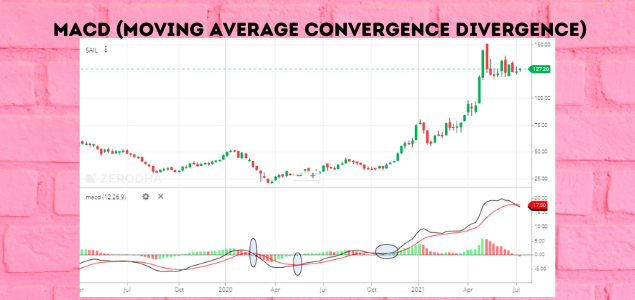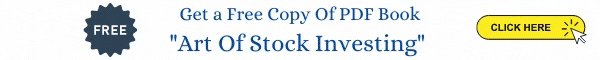##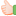Technical Indicators for Beginners

Technical indicators are outcome of some mathematical calculations using Price, Volume, Time etc. Traders use technical indicators to identify different information which will assist in trading trading decisions. As the name suggests, the indicators indicate where the price can go next.

Indicators provide the following information for traders:

 Trend
Using some of the indicators, traders can identify the trend of the market or the direction in which the market is moving. Typically, the trend indicators are oscillators, they tend to move between high and low values. Commonly used indicator is moving average.

 Momentum
Momentum indicators indicate the strength of the trend and indicates whether there is any likelihood of reversal. Relative Strength Index (RSI) is one momentum indicator, it is used for indicating the overbought and oversold levels. MACD, ADX are some of the momentum indicators.

 Volume
Volume indicators show how the volume changes with time and also the strength of the move. On-Balance Volume, Money Flow Index are some of the volume indicators.

 Volatility
Volatility is one of the most important indicators, it indicates how much the price is changing in the given period. Bollinger Bands and ATR (Average True range) are some of the volatility indicators.

Widely used Technical Indicators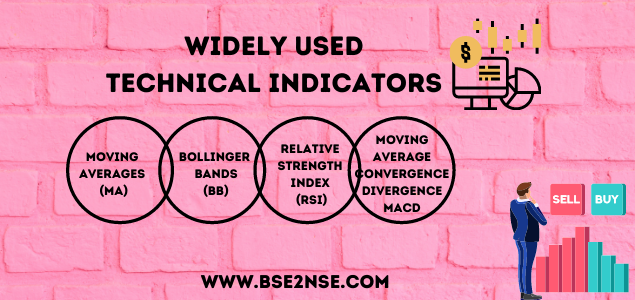Moving Averages (MA)

Moving averages is a frequently used indicators. It provides information about the momentum of the market, trends in the market, the reversal of trends, and the stop loss and stop-loss points. Moving average allows the traders to find out the trading opportunities in the direction of the current market trend.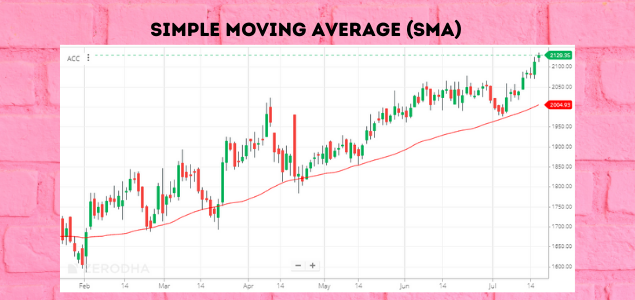Exponential Moving Average

This is the more advanced and more trusted form of moving average. The main difference between EMA and SMA is the weightage given to values. In a simple moving average, all the values are given equal weightage. But in the case of Exponential Moving Average, the more recent values are given more weightage.

EMA is quicker to react to the current market price because EMA gives more importance to the most recent data points. This helps the trader to take quicker trading decisions. Hence, for this reason, traders prefer the use of the EMA over the SMA.

Some use combination of EMA to know the trend.
Up Trend  9EMA > 20EMA.
Down Trend  9EMA < 20EMA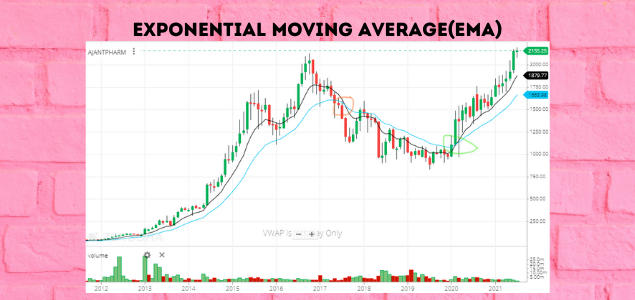Bollinger Bands

Bollinger bands indicate the volatility in the market. Bollinger bands consists of three components,
 a middle line which is a 20-day simple moving average,
 a +2 standard deviation upper bang and
 a -2 lower deviation lower band.

The price of a stock moves between the upper and the lower band. When the market is moving and the volatility is greater, the band widen and when the volatility is less the gap decreases. Bollinger bands help traders to understand the price range of a particular stock.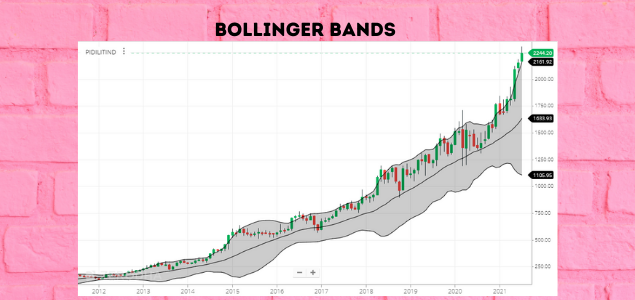Relative Strength Index (RSI)

Relative Strength Index (RSI) is a momentum indicator. It is a single line ranging from 0 to 100 which indicates when the stock is overbought or oversold in the market. If the reading is above 70, it indicates an overbought market and if the reading is below 30, it is an oversold market. RSI is also used to estimate the trend of the market, if RSI is above 50, the market is considered to be in uptrend and if the RSI is below 50, the market is considered to be in downtrend. These values change from individual to individual. Some traders use 20-80 range. Some traders use 60 as the cut-off for trend identification.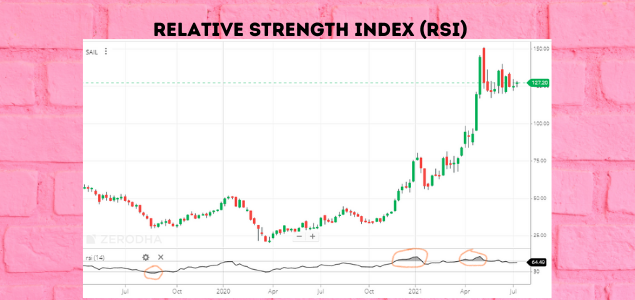MACD (Moving Average Convergence Divergence)

MACD is an Acronym for Moving Average Convergence and Divergence. As the name would suggest, MACD is a convergence and divergence of two moving averages. Therefore, convergence here is the movement of two moving averages towards each other. And divergence is the movement of two moving averages away from each other.
MACD is calculated by using a 12 day EMA and 26 EMA. The convergence or divergence is calculated by subtracting 26 EMA from 12 EMA. And a simple line graph is plotted from the calculated values and it is called a MACD line.

If the 12 EMA is higher than 26 EMA, it means that there is positive momentum in the market because the short-term EMA is a better indicator of current market strength. If the 12 EMA is below 26 EMA, that means that there is negative momentum in the market.

The difference between the two EMA is the MACD spread. When the share/stock is in momentum, then the spread increases. And the spread decreases when the momentum dwindles. We should look for buying opportunities when the spread is positive and vice-versa when the spread is negative.